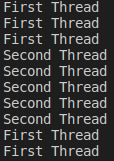# Methods of Thread class in Python

## Methods of Thread class in Python

1. active_count()
2. enumerate()
3. isAlive()
4. join()
5. join(seconds)
##### active_count() method:

This function returns the number of active threads currently running.

Program: active_count() method of Thread class in Python (demo9.py)

```from threading import *
import time

def display():
time.sleep(3)

print("The Number of active Threads:", active_count())
t1.start()
t2.start()
t3.start()
print("The Number of active Threads:", active_count())
time.sleep(5)
print("The Number of active Threads:", active_count())```

Output: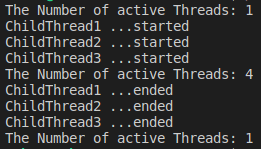Initially, the number of active threads is only the Main thread. Later, when we created three threads to the target, then the total thread would become four.

##### enumerate() function:

This function returns a list of the objects of all active threads currently running.

Program: enumerate() function of Thread class (demo10.py)

```from threading import *
import time

def display():
time.sleep(3)

t1.start()
t2.start()
t3.start()

l=enumerate()
for t in l:

time.sleep(5)
l=enumerate()
for t in l:

Output: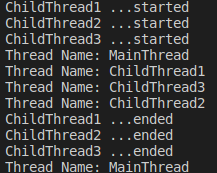After starting the display() function to run in three threads, a total of three plus one main threads would be running at the same time. After three seconds, the sleep time, the child threads would end. So, after that time, only the main thread would be in the execution.

##### isAlive() method:

This method is used to check whether a thread is still executing or not.

Program: isAlive() method in Python (demo11.py)

```from threading import *
import time

def display():
time.sleep(3)

print("The Number of active Threads:", active_count())

t1.start()
t2.start()

print(t1.name,"is Alive :",t1.isAlive())
print(t2.name,"is Alive :",t2.isAlive())

time.sleep(5)

print(t1.name,"is Alive :",t1.isAlive())
print(t2.name,"is Alive :",t2.isAlive())```

Output: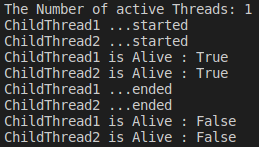##### join() method:

If we want a particular thread to wait for another thread to complete, then we can use join() method.

Program: join() method of Thread class (demo12.py)

```from threading import *
import time

def display():
for i in range(5):
t.start()
t.join() #This is executed by Main Thread

for i in range(5):

Output: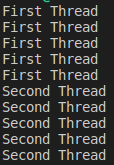Here the main thread will wait until the child thread gets completed.

##### join(seconds) method:

The join method above can also be used by passing the argument ,in the seconds, time period also. In this case thread will wait only specified amount of time.

Program: join(seconds) method in Python (demo13.py)

```from threading import *
import time

def display():
for i in range(5):